# Welcome

Welcome to the EMDomics package! This vignette will explain the functionality of the package through the creation and analysis of a toy data set.

# Earth Mover’s Distance

EMDomics analyzes differences in genomics data between groups of samples. Typically the data will be gene expression levels from array- or sequence-based experiments, but other scenarios are possible. In a real two-class experiment, the groups might be test vs. control, sensitive vs. resistant, etc. In a multi-class experiment (i.e., more than two groups of patients), groups may be associated with patients (in the case of single cell measurements) or disease subtypes. Typically you’ll be analyzing differences across multiple genes, but we’ll start with a single gene to get a feel for how the Earth Mover’s Distance (EMD) algorithm works. Note also that this package includes functionality for Komolgorov-Smirnov (K-S) and Cramer von Mises (CVM) distribution comparison tests. To access these tests, use calculate_ks or calculate_cvm. The input and output syntax is the same as calculate_emd, with “emd” being replaced with “ks” or “cvm” when accessing output values.

Because this package is EMDomics we will go through functionality with calculations for EMD, but K-S and CVM can be accessed with ease by replacing the function name.

We’ll create a vector of expression data for 100 samples. We’ll assign the first 50 to group “A,” the next 20 to group “B,” and the final 30 to group “C.” We will create a vector of group labels that describes which group each of the samples is a part of. Note that the vector of labels must have names corresponding to the sample identifiers in the data:

exp_data <- rnorm(100)
names(exp_data) <- paste("sample", 1:100)

groupA.labels <- rep("A",50)
groupB.labels <- rep("B",20)
groupC.labels <- rep("C",30)

labels <- c(groupA.labels, groupB.labels, groupC.labels)
names(labels) <- names(exp_data)

We’ll take a quick look at the three distributions using ggplot:

library(ggplot2)

df <- as.data.frame(exp_data)
df$group[1:50] <- "A" df$group[51:70] <- "B"
df$group[71:100] <- "C" ggplot(df, aes(exp_data, fill=group)) + geom_density(alpha=0.5)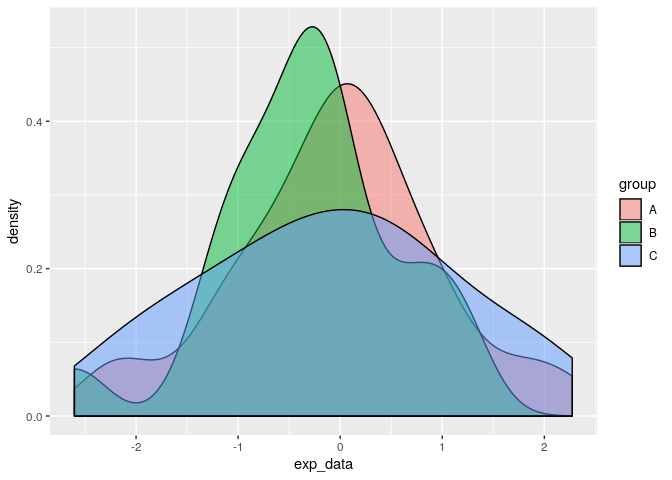We shouldn’t expect the three groups to look too different, since we’re just sampling from the normal distribution. Intuitively, the “work” required to transform any one distribution into another should be low. We can calculate the EMD score for this single gene using the function calculate_emd_gene: library(EMDomics) calculate_emd_gene(exp_data, labels, names(exp_data)) ##  2.2 Now we’ll modify the expression data for group A and see how the EMD score changes. We’ll randomly add or subtract 2 from each data point in group A: exp_data2 <- exp_data mod_vec <- sample(c(2,-2), 50, replace=TRUE) exp_data2[1:50] <- exp_data2[1:50] + mod_vec Let’s again visualize the distributions and calculate the EMD score: df <- as.data.frame(exp_data2) df$group[1:50] <- "A"
df$group[51:70] <- "B" df$group[71:100] <- "C"

ggplot(df, aes(exp_data2, fill=group)) + geom_density(alpha=0.5)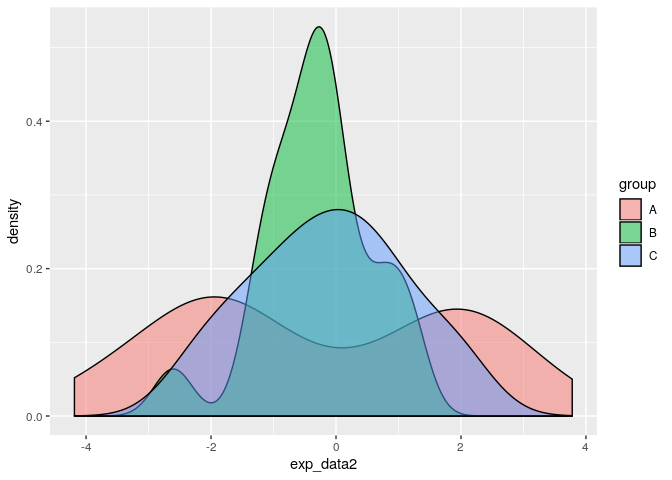calculate_emd_gene(exp_data2, labels, names(exp_data2))
##  6.82

The EMD score is larger, reflecting the increased work needed to transform one distribution into another. Note that since we have three classes defined, we aren’t able to tell from the EMD score alone which two groups (or, potentially, all three groups) demonstrate differences in gene behavior. The composite EMD score in a multi-class analysis is the average of all the pairwise EMD scores. The pairwise EMD scores are computed by comparing all possible combinations of two of the classes. More information on multi-class analysis is in the next section.

Note that in a two-class analysis, a greater EMD score is directly indicative of a greater difference between the measurement distributions of the two classes.

# Analyzing Significance

The EMD score increases as the distributions become increasingly dissimilar, but we have no framework for estimating the significance of a particular EMD score. EMDomics uses a permutation-based method to calculate a q-value that is interpreted analogously to a p-value. To access the full functionality of the package, we’ll use the function calculate_emd.

We’ll first create a matrix of gene expression data for 100 samples (tumors, patients, etc.) and 100 genes. We’ll just sample from the normal distribution for now. The first 50 samples will be our “group A,” second 20 will be “group B,” and the final 30 will be “group C.” Just as before, we will store these sample labels in a named vector associating group with sample identifier:

data <- matrix(rnorm(10000), nrow=100, ncol=100)
rownames(data) <- paste("gene", 1:100, sep="")
colnames(data) <- paste("sample", 1:100, sep="")

groupA.labels <- rep("A",50)
groupB.labels <- rep("B",20)
groupC.labels <- rep("C",30)

labels <- c(groupA.labels, groupB.labels, groupC.labels)
names(labels) <- colnames(data)

Now we can call calculate_emd. We’ll only use 10 permutations for the purposes of this vignette, but in actual experiments using at least 100 permutations is advised. For this example we will turn off parallel processing, but in general it should be enabled.

results <- calculate_emd(data, labels, nperm=10, parallel=FALSE)

Most of the time, you’ll be interested in the emd matrix returned as a member of the return object:

emd <- results$emd head(emd) ## emd q-value ## gene1 1.880000 1.0000000 ## gene2 2.116667 0.5806452 ## gene3 1.716667 1.0000000 ## gene4 2.120000 0.5806452 ## gene5 2.450000 0.1500000 ## gene6 3.060000 0.0000000 This matrix lists the emd score and the q-value for each gene in the data set. Because we’re not analyzing many genes and the data is randomly generated, there may be some significant q-values in the results simply by chance. We can order the emd matrix by q-value: emd2 <- emd[(order(emd[,"q-value"])),] head(emd2) ## emd q-value ## gene6 3.060000 0 ## gene13 3.110000 0 ## gene31 3.283333 0 ## gene37 3.000000 0 ## gene38 4.866666 0 ## gene48 3.026667 0 Note the correlation of significant q-values with relatively large EMD scores. In a multi-class analysis, it may not be enough to know that a gene behaves differently somehow among the defined classes. We may be interested in finding which two classes display a greater difference in gene behavior, or if all three classes are somehow different. The differences between each of the classes is defined in the pairwise.emd.table. Note that EMD is not directional, so all possible combinations, not permutations, of the class labels are used in the pairwise EMD score calculations. Each of the columns represents a pairwise comparison (e.g. Group A vs Group B), each row represents a gene, and the cell content is the EMD score quantifying the work required to transform the distribution of one group into the other. emd.pairwise <- results$pairwise.emd.table
head(emd.pairwise)
##       A vs B    A vs C    B vs C
## gene1   1.78 1.8800000 1.0666666
## gene2   1.61 1.0066668 2.1166666
## gene3   1.55 1.0133333 1.7166666
## gene4   2.12 1.2066667 1.4000001
## gene5   2.45 2.1800001 0.7500001
## gene6   3.06 0.9333333 2.5333333

# Visualization

EMDomics includes a few visualization functions. The function plot_density will display the density distributions of each of the groups for a given gene, along with the EMD score. We can compare the gene with the largest EMD score and the gene with the smallest EMD score, for example:

emd3 <- emd[(order(emd[,"emd"])),]
smallest_gene <- rownames(emd3)
biggest_gene <- rownames(emd3)[nrow(emd3)]

plot_emd_density(results, smallest_gene)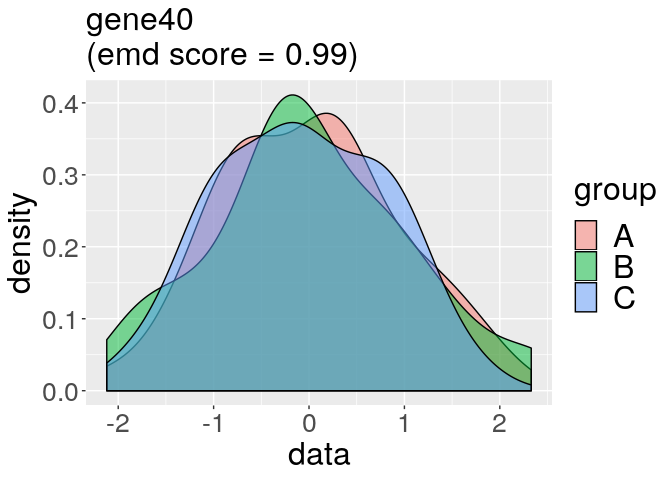plot_emd_density(results, biggest_gene)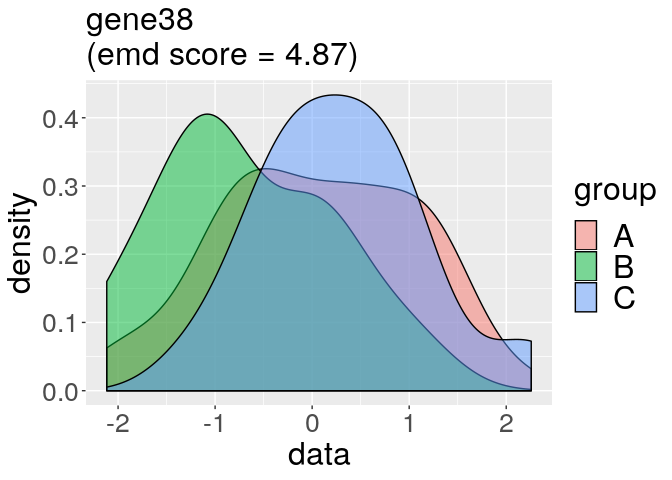Note that the EMD score is the average of the each of the pairwise EMD scores. This means that the smallest and largest EMD scores may have ambiguous meanings in a multi-class analysis. To understand how each class compares to the others, the pairwise.emd.table provides pairwise comparisons of gene behavior. These pairwise EMD scores will lend more insight into how the gene is similar or different across classes.

In a two-class analysis, the smallest score represents the gene that demonstrates the most similar behavior in both classes, and the largest score represents the gene that demonstrates the most different behavior in both classes.

We can plot a histogram of all the calculated EMD scores with the function plot_emdperms:

plot_emdperms(results)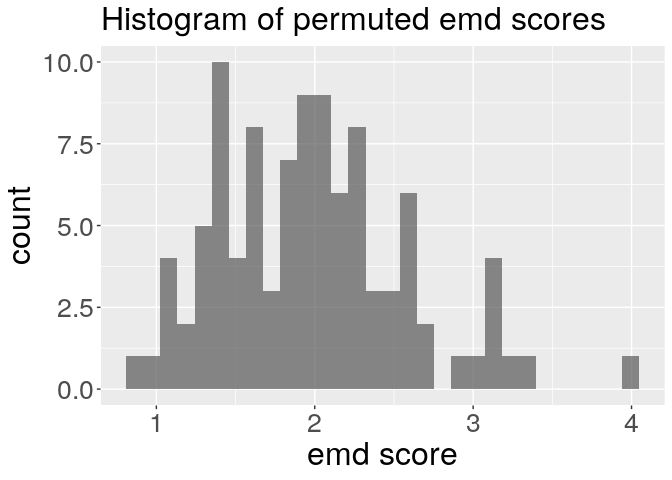This plot can help intuitively understand the relative significance of an EMD score. For example, almost all the randomly permuted EMD scores are smaller than the largest calculated EMD score plotted above.

In a similar vein, the function plot_emdnull plots the null distribution (the median of the permuted EMD scores) for each gene vs. the calculated EMD score (the line x=y is superimposed in red):

plot_emdnull(results)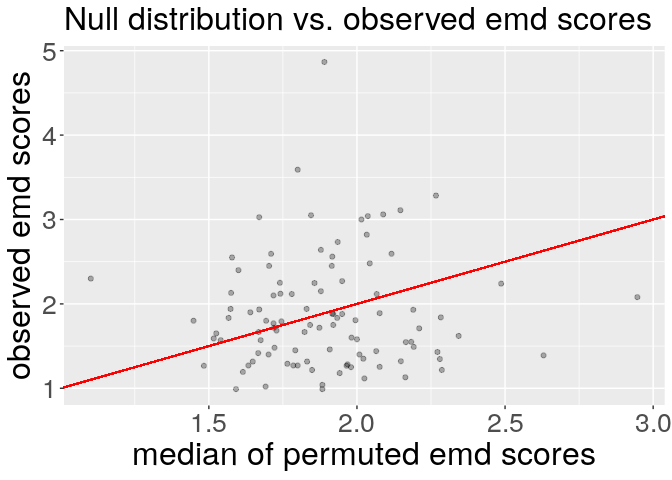# Wrapping Up

This concludes the EMDomics vignette. For additional information, please consult the reference manual.

# Session Info

## R version 3.6.1 (2019-07-05)
## Platform: x86_64-pc-linux-gnu (64-bit)
## Running under: Ubuntu 18.04.3 LTS
##
## Matrix products: default
## BLAS:   /home/biocbuild/bbs-3.10-bioc/R/lib/libRblas.so
## LAPACK: /home/biocbuild/bbs-3.10-bioc/R/lib/libRlapack.so
##
## locale:
##   LC_CTYPE=en_US.UTF-8       LC_NUMERIC=C
##   LC_TIME=en_US.UTF-8        LC_COLLATE=C
##   LC_MONETARY=en_US.UTF-8    LC_MESSAGES=en_US.UTF-8
##   LC_PAPER=en_US.UTF-8       LC_NAME=C
##  LC_MEASUREMENT=en_US.UTF-8 LC_IDENTIFICATION=C
##
## attached base packages:
##  stats     graphics  grDevices utils     datasets  methods   base
##
## other attached packages:
##  EMDomics_2.16.0 ggplot2_3.2.1
##
## loaded via a namespace (and not attached):
##   Rcpp_1.0.2            pillar_1.4.2          compiler_3.6.1
##   tools_3.6.1           emdist_0.3-1          digest_0.6.22
##   preprocessCore_1.48.0 evaluate_0.14         tibble_2.1.3
##  gtable_0.3.0          pkgconfig_2.0.3       rlang_0.4.1
##  yaml_2.2.0            parallel_3.6.1        xfun_0.10
##  withr_2.1.2           dplyr_0.8.3           stringr_1.4.0
##  knitr_1.25            grid_3.6.1            tidyselect_0.2.5
##  glue_1.3.1            R6_2.4.0              BiocParallel_1.20.0
##  rmarkdown_1.16        purrr_0.3.3           magrittr_1.5
##  scales_1.0.0          htmltools_0.4.0       matrixStats_0.55.0
##  CDFt_1.0.1            assertthat_0.2.1      colorspace_1.4-1
##  labeling_0.3          stringi_1.4.3         lazyeval_0.2.2
##  munsell_0.5.0         crayon_1.3.4